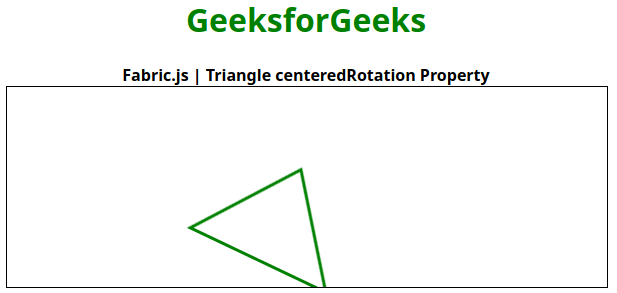# Fabric.js | Triangle centeredRotation Property

• Last Updated : 02 Feb, 2021

In this article, we are going to see how to disable the centered rotation of the canvas triangle using FabricJS. The canvas triangle means the triangle is movable and can be stretched according to requirement. Further, the triangle can be customized when it comes to initial stroke color, height, width, fill color, or stroke width.

To make this possible we are going to use a JavaScript library called FabricJS. After importing the library, we will create a canvas block in the body tag which will contain the triangle. After this, we will initialize instances of Canvas and triangle provided by FabricJS and disable the centered rotation of the canvas triangle using centeredRotation property and render the triangle on the Canvas as given in the example below.

Syntax:

```fabric.Triangle({
width: number,
height: number,
centeredRotation: boolean
}); ```

Parameters: This function accepts three parameters as mentioned above and described below:

• width: This parameter defines the width of triangle.
• height: This parameter defines the height of triangle.
• centeredRotation: This parameter defines whether to enable or disable centered rotation.

Example: This example uses FabricJS to disable the centered rotation of canvas-like triangle as given below. Try rotating the object after disabling centered rotation, it will use the upper-left corner as a center of rotation.

## HTML

 ```<``html``>` `<``head``>``    ``<``title``>``        ``Fabric.js | Triangle centeredRotation Property``    ````    ` `    ````    ``<``script` `src``=``"https://cdnjs.cloudflare.com/ajax/libs/fabric.js/3.6.2/fabric.min.js"``>``    ````` `<``body``>``        ``<``div` `style``=``"text-align: center;width: 600px;"``>``        ``<``h1` `style``=``"color: green;"``>``            ``GeeksforGeeks``        ````        ``<``b``>``            ``Fabric.js | Triangle centeredRotation Property``        ````        ````    ``<``canvas` `id``=``"canvas"` `width``=``"600"` `height``=``"200"``        ``style``=``"border:1px solid #000000"``>``    ````    ` `    ``<``script``>``    ` `        ``// Initiate a Canvas instance``        ``var canvas = new fabric.Canvas("canvas");` `        ``// Initiate a triangle instance``        ``var triangle = new fabric.Triangle({``                        ``width: 150,``                        ``height: 100,``                        ``fill: '',``                        ``stroke: 'green',``                        ``strokeWidth: 3,``                        ``cornerColor: 'blue',``                        ``centeredRotation: false``        ``});` `        ``// Render the triangle in canvas``        ``canvas.add(triangle);``        ``canvas.centerObject(triangle);``    ````` ``

Output:My Personal Notes arrow_drop_up